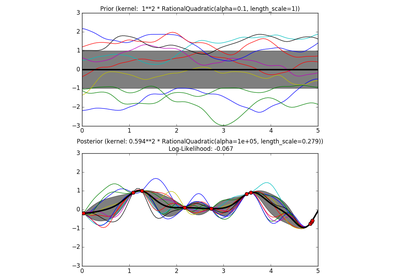# `sklearn.gaussian_process.kernels`.Matern¶

class `sklearn.gaussian_process.kernels.``Matern`(length_scale=1.0, length_scale_bounds=(1e-05, 100000.0), nu=1.5)[source]

Matern kernel.

The class of Matern kernels is a generalization of the RBF and the absolute exponential kernel parameterized by an additional parameter nu. The smaller nu, the less smooth the approximated function is. For nu=inf, the kernel becomes equivalent to the RBF kernel and for nu=0.5 to the absolute exponential kernel. Important intermediate values are nu=1.5 (once differentiable functions) and nu=2.5 (twice differentiable functions).

See Rasmussen and Williams 2006, pp84 for details regarding the different variants of the Matern kernel.

New in version 0.18.

Parameters: length_scale : float or array with shape (n_features,), default: 1.0 The length scale of the kernel. If a float, an isotropic kernel is used. If an array, an anisotropic kernel is used where each dimension of l defines the length-scale of the respective feature dimension. length_scale_bounds : pair of floats >= 0, default: (1e-5, 1e5) The lower and upper bound on length_scale nu: float, default: 1.5 : The parameter nu controlling the smoothness of the learned function. The smaller nu, the less smooth the approximated function is. For nu=inf, the kernel becomes equivalent to the RBF kernel and for nu=0.5 to the absolute exponential kernel. Important intermediate values are nu=1.5 (once differentiable functions) and nu=2.5 (twice differentiable functions). Note that values of nu not in [0.5, 1.5, 2.5, inf] incur a considerably higher computational cost (appr. 10 times higher) since they require to evaluate the modified Bessel function. Furthermore, in contrast to l, nu is kept fixed to its initial value and not optimized.

Methods

 `clone_with_theta`(theta) Returns a clone of self with given hyperparameters theta. `diag`(X) Returns the diagonal of the kernel k(X, X). `get_params`([deep]) Get parameters of this kernel. `is_stationary`() Returns whether the kernel is stationary. `set_params`(\*\*params) Set the parameters of this kernel.
`__init__`(length_scale=1.0, length_scale_bounds=(1e-05, 100000.0), nu=1.5)[source]
`bounds`

Returns the log-transformed bounds on the theta.

Returns: bounds : array, shape (n_dims, 2) The log-transformed bounds on the kernel’s hyperparameters theta
`clone_with_theta`(theta)[source]

Returns a clone of self with given hyperparameters theta.

`diag`(X)[source]

Returns the diagonal of the kernel k(X, X).

The result of this method is identical to np.diag(self(X)); however, it can be evaluated more efficiently since only the diagonal is evaluated.

Parameters: X : array, shape (n_samples_X, n_features) Left argument of the returned kernel k(X, Y) K_diag : array, shape (n_samples_X,) Diagonal of kernel k(X, X)
`get_params`(deep=True)[source]

Get parameters of this kernel.

Parameters: deep: boolean, optional : If True, will return the parameters for this estimator and contained subobjects that are estimators. params : mapping of string to any Parameter names mapped to their values.
`hyperparameters`

Returns a list of all hyperparameter specifications.

`is_stationary`()[source]

Returns whether the kernel is stationary.

`n_dims`

Returns the number of non-fixed hyperparameters of the kernel.

`set_params`(**params)[source]

Set the parameters of this kernel.

The method works on simple kernels as well as on nested kernels. The latter have parameters of the form `<component>__<parameter>` so that it’s possible to update each component of a nested object.

Returns: self :
`theta`

Returns the (flattened, log-transformed) non-fixed hyperparameters.

Note that theta are typically the log-transformed values of the kernel’s hyperparameters as this representation of the search space is more amenable for hyperparameter search, as hyperparameters like length-scales naturally live on a log-scale.

Returns: theta : array, shape (n_dims,) The non-fixed, log-transformed hyperparameters of the kernel

## Examples using `sklearn.gaussian_process.kernels.Matern`¶Illustration of prior and posterior Gaussian process for different kernels